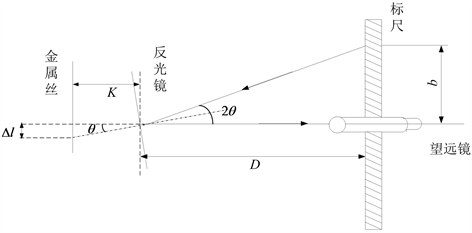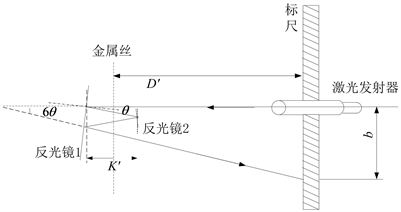# 杨氏模量测量误差研究及实验仪器的改进Research on Young’s Modulus Measurement Error and Improvement of Experimental Instruments

DOI: 10.12677/APP.2019.911055, PDF, HTML, XML, 下载: 348  浏览: 2,302

Abstract: Through the analysis of the principle and data error of the Young’s modulus of wire, several key factors affecting the experimental error are studied, and a design scheme to reduce the experi-mental error is proposed. The experimental equipment is improved by increasing the number of optical levers to enlarge the magnification; fixing the relative position between the experimental instruments and reducing the unstable factors of the measurement. The experimental measure-ment using the improved experimental device can significantly reduce the uncertainty and improve the reliability of the measured value.

1. 引言

2. 实验原理Figure 1. Experimental principle of Young’s modulus measurement

$\theta \approx \mathrm{tan}\theta =\frac{\Delta l}{K}$ (1)

$\mathrm{tan}2\theta \approx 2\theta =\frac{b}{D}$ (2)

$\Delta l=\frac{Kb}{2D}$ (3)

$Y=\frac{Fl}{S\Delta l}$ (4)

3. 实验存在的误差分析

3.1. 仪器误差分析

3.2. 实验操作的误差

4. 设计方案

4.1. 实验装置的改进方案Figure 2. Experimental schematic diagram of the improved device

$\theta \approx \mathrm{tan}\theta =\frac{\Delta l}{K}$ (5)

$\mathrm{tan}6\theta \approx 6\theta =\frac{b}{{D}^{\prime }}$ (6)

$\Delta l=\frac{{K}^{\prime }b}{12{D}^{\prime }}$ (7)

$Y=\frac{Fl}{S\Delta l}=\frac{12Fl{D}^{\prime }}{\pi {d}^{2}{K}^{\prime }b}$ (8)

4.2. 实验操作方法的改进

4.3. 实验操作方法的改进Table 1. Measurement data of wire diameterTable 2. Measurement data of scale reading before device improvementTable 3. Measurement data of scale reading after improvement of the device

5. 结论

  秦先明. 拉伸法测杨氏模量的实验误差分析及其消除方法[J]. 中国科学教育, 2005(1): 91-92.  周晓明. 三种杨氏模量测量方法比较[J]. 实验科学与技术, 2011, 9(6): 97-99.  张由群. 杨氏弹性模量的研究[J]. 实验科学与技术, 2004, 3(1): 82-84.  朱博. 杨氏弹性模量测量仪的改进[J]. 大学物理, 2008, 27(3): 43-44.  高允锋, 李鸿英, 韩飞. 杨氏模量测定及误差计算[J]. 长春师范学院学报, 2008, 27(2): 39-42.  李书义, 宋金璠. 杨氏模量测定实验误差分析与研究[J]. 南阳师范学院学报, 2008, 7(3): 35-37.  穆晓东. 游标卡尺在测定金属丝杨氏模量中的应用[J]. 大学物理实验, 2013, 26(4): 63-64.  李霞, 赵树民, 贾鹏英, 等. 光杠杆原理在钢丝杨氏模量测定中的近似[J]. 物理通报, 2007(3): 41-43.  李儒颂, 叶文江. 金属丝杨氏模量测量装置的设计[J]. 大学物理实验, 2014, 27(5): 51-53.  徐庆君, 刘伟, 贾国治. 金属丝的杨氏模量测定实验的改进[J]. 物理实验, 2007, 27(9): 40-41.  高德文. 杨氏模量试验中不确定度的评定方法[J]. 大学物理, 2005, 24(6): 44-46.  李远兴. 杨氏模量实验的不确定度评定[J]. 四川兵工学报, 2009, 30(7): 137-138.  庄建. 关于实验“拉伸法测杨氏模量”的思考[J]. 实验科学与技术, 2006, 4(3): 69-71.  赵敏, 强晓明, 章韦芳. 杨氏模量测定实验的整体改进[J]. 大学物理, 2012, 31(7): 37-39.  杨桂娟, 梅妍. 大学物理实验[M]. 大连: 大连理工大学出版社, 2006: 54-58.